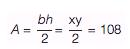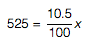Can you ace these GRE math practice questions? Start honing your skills with some GRE math practice and get a preview of what you can expect on test day.

We pulled these GRE quantitative practice questions from our book Cracking the GRE and from our GRE prep course  materials. Each sample question includes an explanation, so you can see how to crack it!

Note about calculators: On the GRE you'll be given an on-screen calculator with the five basic operations: addition, subtraction, multiplication, division and square root, plus a decimal function and positive/negative feature. Don't use anything fancier when you tackle this GRE math practice!

## Practice for GRE Quantitative Comparison Questions

Quantitative comparison questions ask you to compare Quantity A to Quantity B. Your job is to compare the two quantities and decide which of the following describes the relationship:

• Quantity A is greater.
• Quantity B is greater.
• The two quantities are equal.
• The relationship cannot be determined from the information given.

1. The average (arithmetic mean) high temperature for x days is 70 degrees. The addition of one day with a high temperature of 75 degrees increases the average to 71 degrees.

Quantity A Quantity B
x 5

(A) Quantity A is greater.
(B) Quantity B is greater.
(C) The two quantities are equal.
(D) The relationship cannot be determined from the information given.

Answer: (B) Quantity B is greater.

If the average high temperature for x days is 70 degrees, then the sum of those x high temperatures is 70x. The sum of the high temperatures, including the additional day that has a temperature of 75 degrees is, therefore, 70x + 75. Next, use the average formula to find the value of x:In this formula, 71 is the average, 70x + 75 is the total, and there are x + 1 days. Substituting this information into the formula gives:To solve, cross-multiply to get 71x + 71 = 70x + 75. Next, simplify to find that x = 4. Therefore, Quantity B is greater. The correct answer is (B).

2.
a and b are integers.
a 2 = b 3

Quantity A Quantity B
a b

(A) Quantity A is greater.
(B) Quantity B is greater.
(C) The two quantities are equal.
(D) The relationship cannot be determined from the information given.

Answer: (D) The relationship cannot be determined from the information given.

Try different integers for a and b that satisfy the equation a 2 = b 3 such as a = 8 and b = 3. These numbers satisfy the equation as 8 2 = 4 3 = 64. In this case, Quantity A is greater. Because Quantity B is not always greater nor are the two quantities always equal, choices (B) and (C) can be eliminated. Next, try some different numbers. When choosing a second set of numbers, try something less common such as making a = b = 1. Again, these numbers satisfy the equation provided in the problem. In this case, however, the quantities are equal. Because Quantity A is not always greater, choice (A) can now be eliminated. The correct answer is (D).

## Practice for GRE Multiple-Choice Questions

For question 3, select one answer from the list of five answer choices.

3. A certain pet store sells only dogs and cats. In March, the store sold twice as many dogs as cats. In April, the store sold twice the number of dogs that it sold in March, and three times the number of cats that it sold in March. If the total number of pets the store sold in March and April combined was 500, how many dogs did the store sell in March?

(A) 80
(B) 100
(C) 120
(D) 160
(E) 180

Plug In the Answers, starting with the middle choice. If 120 dogs were sold in March, then 60 cats were sold that month. In April, 240 dogs were sold, along with 180 cats. The total number of dogs and cats sold during those two months is 600, which is too large, so eliminate (C), (D), and (E). Try (B). If there were 100 dogs sold in March, then 50 cats were sold; in April, 200 dogs were sold along with 150 cats. The correct answer is (B) because 100 + 50 + 200 + 150 = 500.

4.ABC has an area of 108 cm 2 . If both x and y are integers, which of the following could be the value of x ?

Indicate all  such values.

(A) 4
(B) 5
(C) 6
(D) 8
(E) 9

Answer: (A), (C), (D) and (E)

Plug the information given into the formula for the area of a triangle to learn more about the relationship between x and y:The product of x and y is 216, so x needs to be a factor of 216. The only number in the answer choices that is not a factor of 216 is 5. The remaining choices are possible values of x.

## Practice for GRE Numeric Entry Questions

Some questions on the GRE won't have answer choices, and you'll have to generate your own answer.

5. Each month, Renaldo earns a commission of 10.5% of his total sales for the month, plus a salary of \$2,500. If Renaldo earns \$3,025 in a certain month, what were his total sales?

If Renaldo earned \$3,025, then his earnings from the commission on his sales are \$3,025 - \$2,500 = \$525. So, \$525 is 10.5% of his sales. Set up an equation to find the total sales:Solving this equation, x = 5,000.

6. At a recent dog show, there were 5 finalists. One of the finalists was awarded "Best in Show" and another finalist was awarded "Honorable Mention." In how many different ways could the two awards be given out?